Test Description

## 15 Questions MCQ Test Mathematics Olympiad for Class 1 | Olympiad Test Level 1: Addition- 2

 1 Crore+ students have signed up on EduRev. Have you?

### Aman scored 16 runs. Mihir scored 12 runs more than Aman. How many runs did they score altogether?

Detailed Solution for Olympiad Test Level 1: Addition- 2 - Question 1

12 more than 16 means to add 12 and 16. So 12 + 16 = 28.
So, both of them scored the runs together = 16 + 28, that is,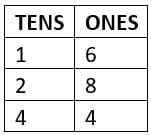### Which abacus shows 1 more than 56?

Detailed Solution for Olympiad Test Level 1: Addition- 2 - Question 2

1 + 56 = 57 = 5 Tens + 7 Ones

### How many eyes do the following animals have in total?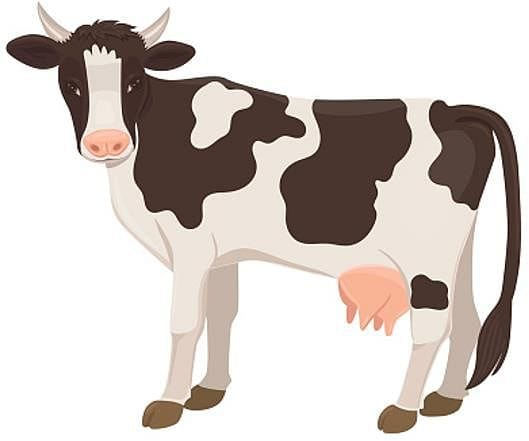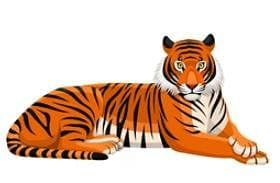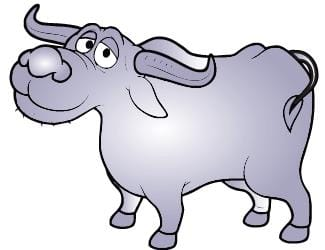Detailed Solution for Olympiad Test Level 1: Addition- 2 - Question 3

Each animal have 2 eyes. So, 2 + 2 + 2 = 6

Which one of the following numbers is equal to 8 more than 7?

Detailed Solution for Olympiad Test Level 1: Addition- 2 - Question 4

7 + 8 = 15

The display of a calculator is given. What number will you get when you press the button =?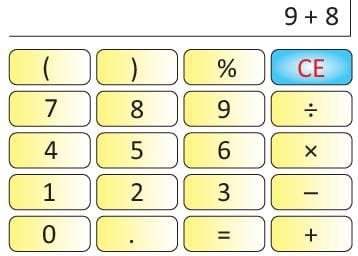Detailed Solution for Olympiad Test Level 1: Addition- 2 - Question 5

9 + 8 = 17.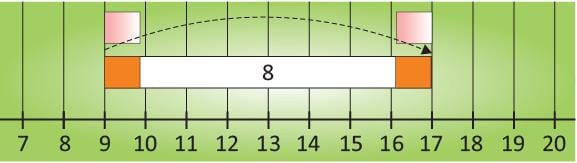Matchbox A has 22 matchsticks Matchbox B has 7 matchsticks. Matchbox C is filled by adding the sticks from A and B. Find the number of matchsticks in C.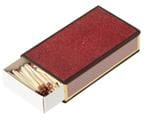Detailed Solution for Olympiad Test Level 1: Addition- 2 - Question 6

22 + 7 = 29

Fill the box.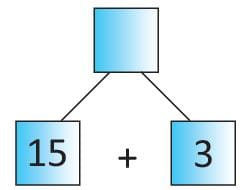Detailed Solution for Olympiad Test Level 1: Addition- 2 - Question 7

15 + 3 = 18

Sheena gave 5 chocolates in class 1 A, 8 chocolates in class 1 B, and 3 chocolates in class 1 C on her birthday. How many chocolates did Sheena distribute in all?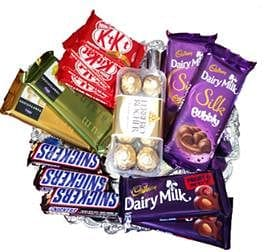Detailed Solution for Olympiad Test Level 1: Addition- 2 - Question 8

5 + 8 + 3 = 16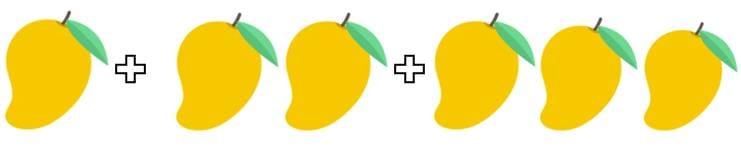Detailed Solution for Olympiad Test Level 1: Addition- 2 - Question 9

1 + 2 + 3 = 6

Payal scored 14 marks in Section A, 5 marks in Section B, 4 marks in Section C, what are the total marks she obtained?

Detailed Solution for Olympiad Test Level 1: Addition- 2 - Question 10

14 + 5 + 4 = 23

Mayur read 32 pages of a book but he still has 7 more pages to read. Find the total number of pages in the book.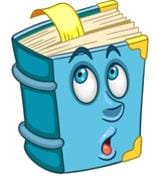Detailed Solution for Olympiad Test Level 1: Addition- 2 - Question 11

32 + 7 = 39

A container contains 11 L of milk. 3 L milk has to be added for filling it completely. What is the capacity of the container?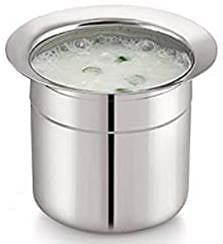Detailed Solution for Olympiad Test Level 1: Addition- 2 - Question 12

11 L + 3 L = 14 L

Which one of the following options will give the sum of 20?

Detailed Solution for Olympiad Test Level 1: Addition- 2 - Question 13

19 + 1 = 20

Karan ate 17 candies but he still has 7 more with him. Find the total number of candies Karan had.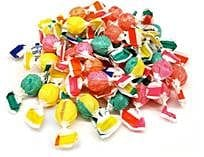Detailed Solution for Olympiad Test Level 1: Addition- 2 - Question 14

17 + 7 = 24

In a calculator, there are 10 number keys and 8 function keys. Find the total number of keys in the calculator.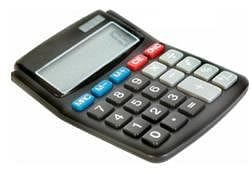Detailed Solution for Olympiad Test Level 1: Addition- 2 - Question 15

10 + 8 = 18

## Mathematics Olympiad for Class 1

9 docs|51 tests
 Use Code STAYHOME200 and get INR 200 additional OFF Use Coupon Code